Ex 12.3

Chapter 12 Class 7 Symmetry
Serial order wise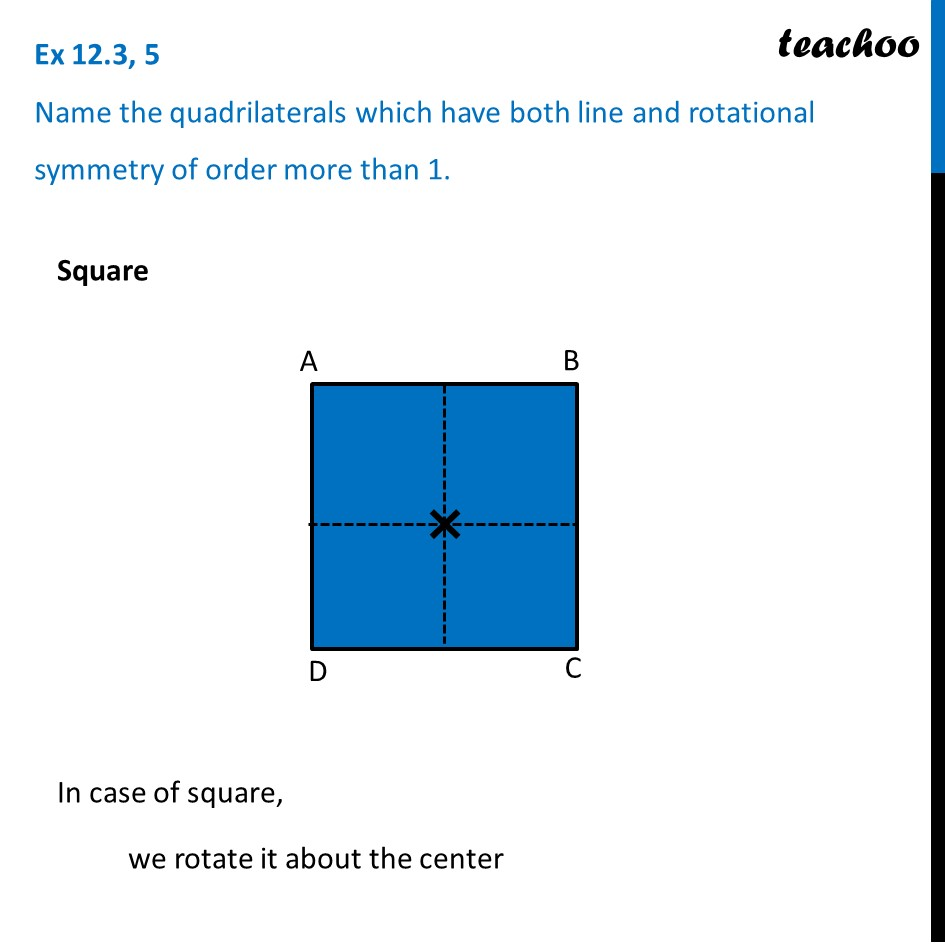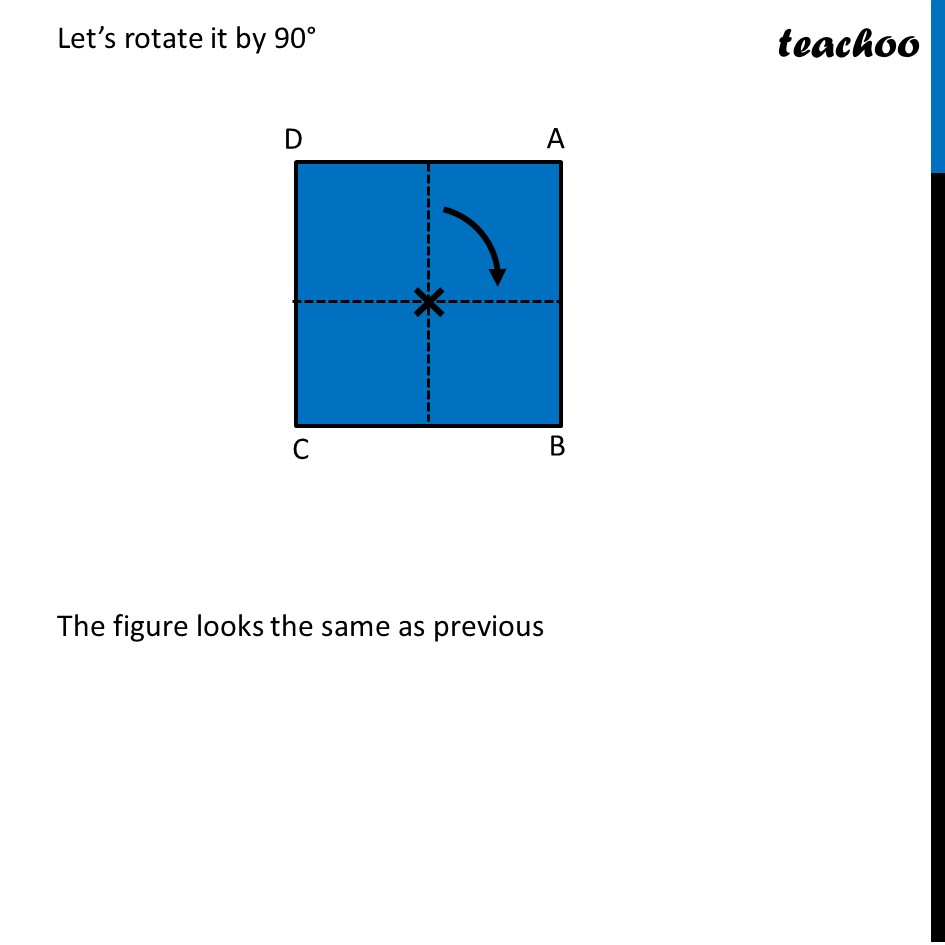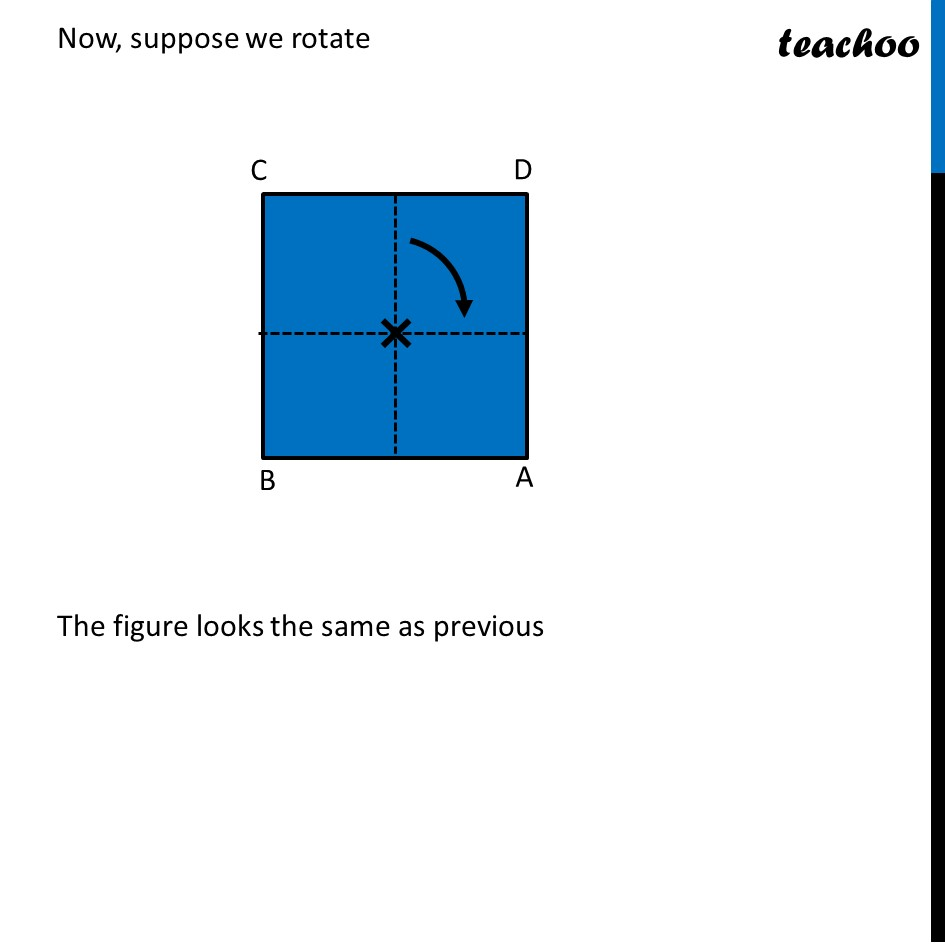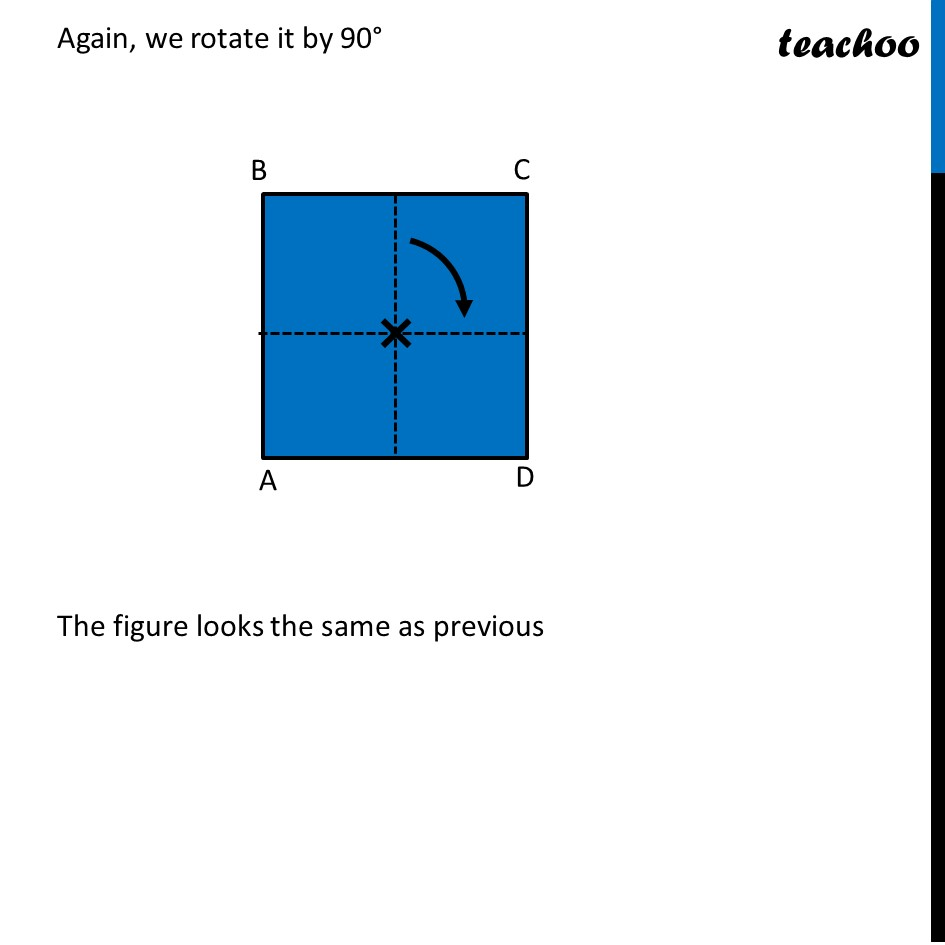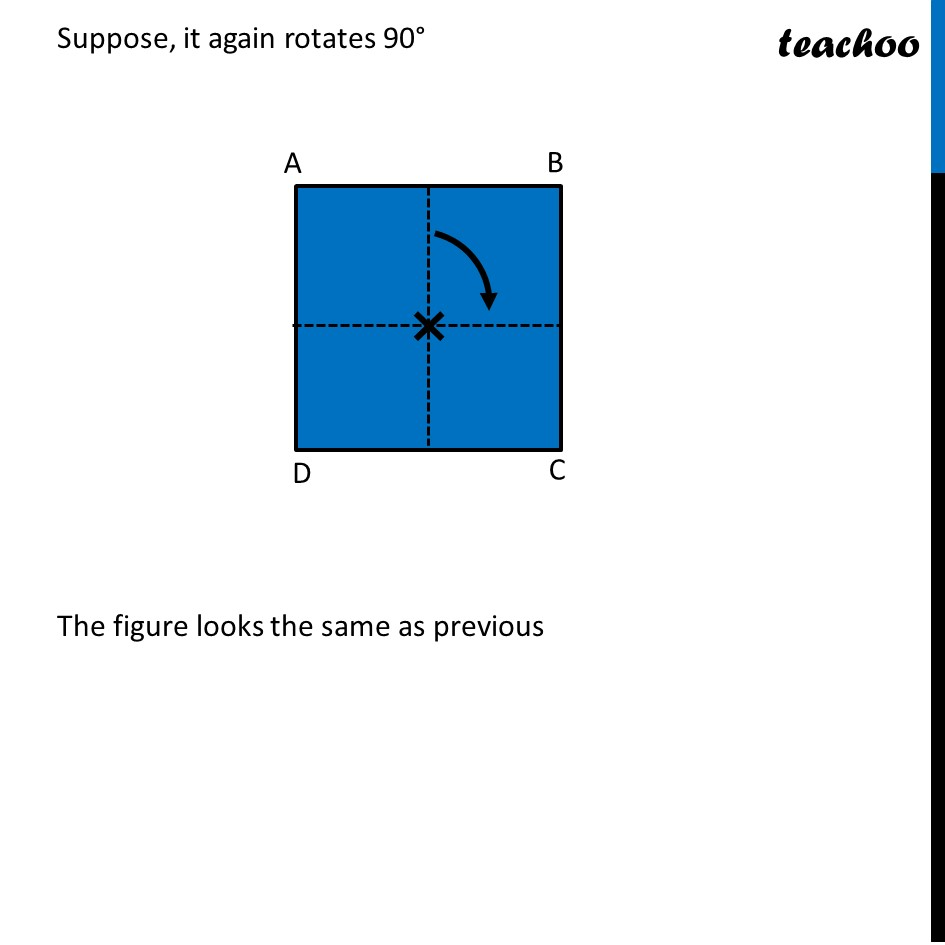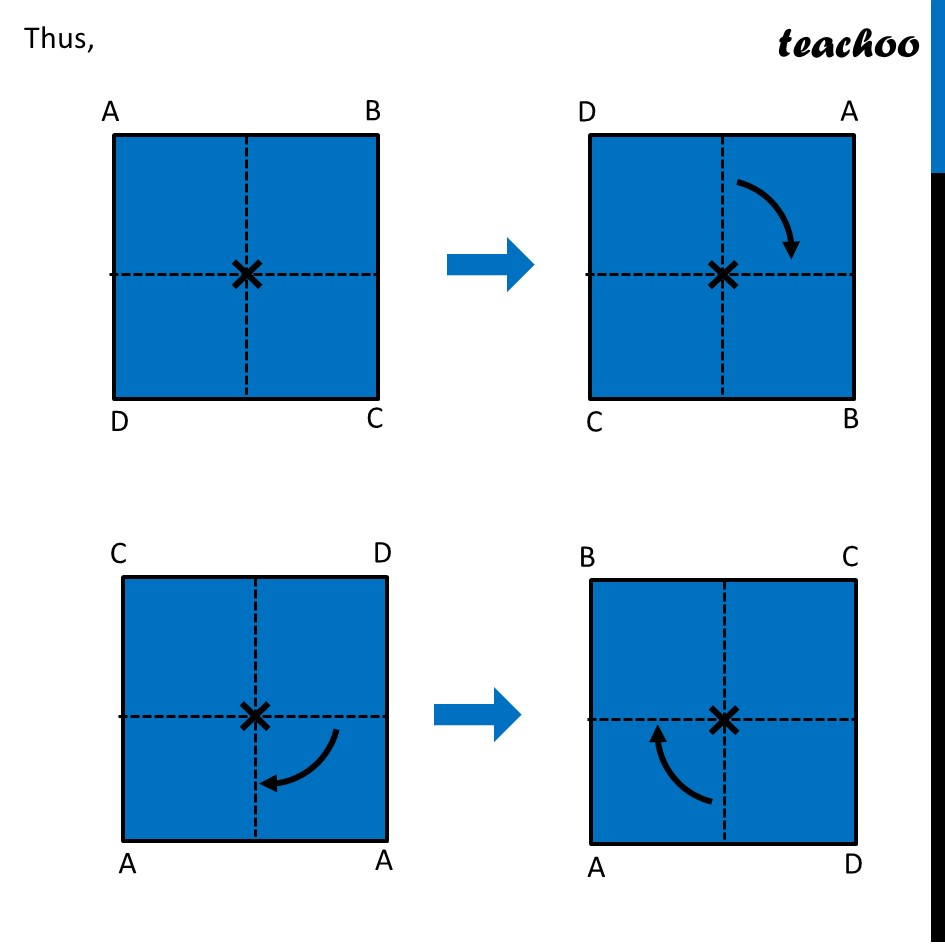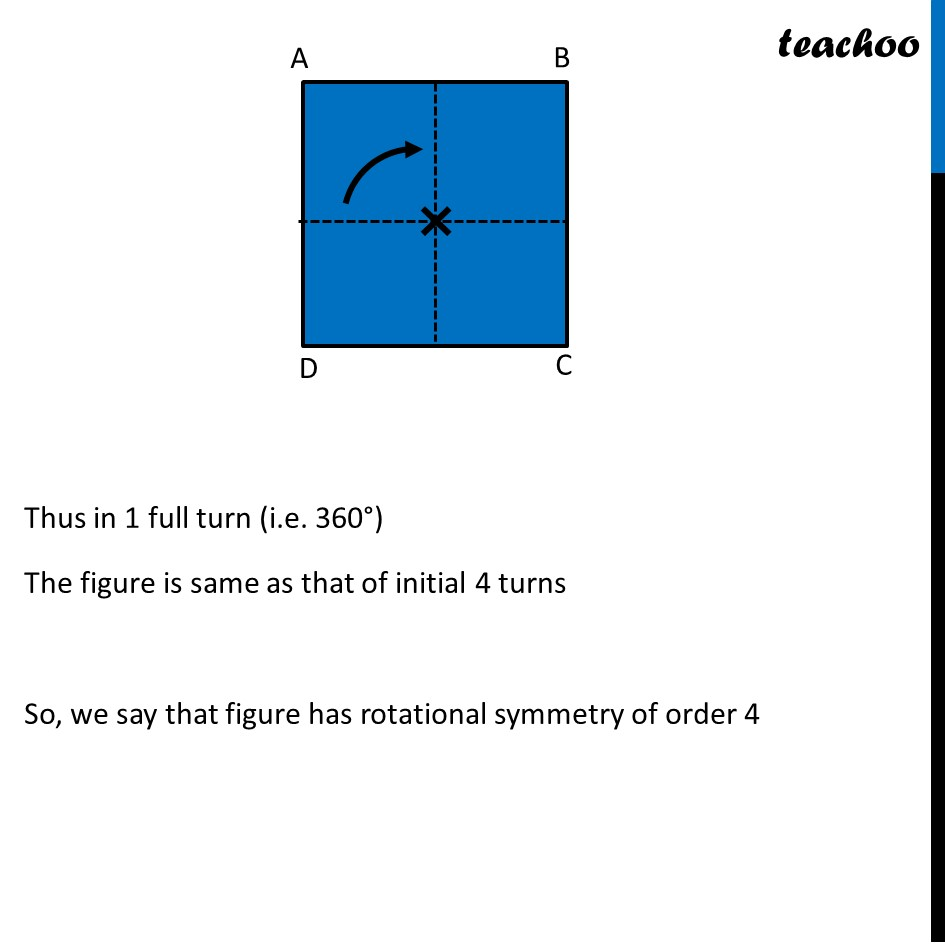Learn in your speed, with individual attention - Teachoo Maths 1-on-1 Class

### Transcript

Ex 12.3, 5 Name the quadrilaterals which have both line and rotational symmetry of order more than 1.Square In case of square, we rotate it about the center Let’s rotate it by 90° The figure looks the same as previous Now, suppose we rotate The figure looks the same as previous Again, we rotate it by 90° The figure looks the same as previous Suppose, it again rotates 90° The figure looks the same as previous Thus, Thus in 1 full turn (i.e. 360°) The figure is same as that of initial 4 turns So, we say that figure has rotational symmetry of order 4